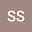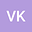loading page

An efficient collocation algorithm with SSP-RK43 to solve Rosenau-KdV-RLW equation
••• Shallu Shallu,
• Vijay Kukreja
Shallu Shallu
Sant Longowal Institute of Engineering and Technology
Author ProfileVijay Kukreja
SLIET, LONGOWALL
Author Profile## Abstract

In this study, coupling of Rosenau-KdV and Rosenau-RLW equation is solved by a collocation technique based on quintic B-spline as basis functions which models the motion of shallow water waves. For this, spatial domain is discretized using quintic B-splines, which leads to a system of first order ordinary differential equations. Strong stability preserving Runge-Kutta method of fourth stage, third-order (SSP-RK43) is applied to solve the obtained system. All the calculations are performed without any linearization or transformation. Couple of test problems are solved to show the efficacy and accuracy of the technique by calculating L2 and L(infinity) error norms as well as discrete energy (E) and mass (Q) conservation properties. Stability analysis of the technique is performed using the concept of Jacobian matrix along with eigenvalues and is shown to be stable.